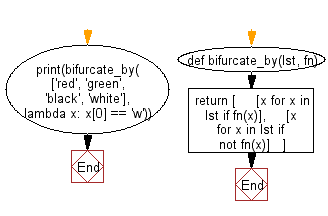﻿ Python: Split values into two groups, based on the result of the given filtering function - w3resource# Python: Split values into two groups, based on the result of the given filtering function

## Python List: Exercise - 217 with Solution

Write a Python program to split values into two groups, based on the result of the given filtering function.

• Use a list comprehension to add elements to groups, based on the value returned by fn for each element.
• If fn returns a truthy value for any element, add it to the first group, otherwise add it to the second group

Sample Solution:

Python Code:

``````def bifurcate_by(lst, fn):
return [
[x for x in lst if fn(x)],
[x for x in lst if not fn(x)]
]
print(bifurcate_by(['red', 'green', 'black', 'white'], lambda x: x == 'w'))
```
```

Sample Output:

```[['white'], ['red', 'green', 'black']]
```

Flowchart:## Visualize Python code execution:

The following tool visualize what the computer is doing step-by-step as it executes the said program:

Python Code Editor:

Have another way to solve this solution? Contribute your code (and comments) through Disqus.

What is the difficulty level of this exercise?

Test your Python skills with w3resource's quiz

﻿

## Python: Tips of the Day

Floor Division:

When we speak of division we normally mean (/) float division operator, this will give a precise result in float format with decimals.

For a rounded integer result there is (//) floor division operator in Python. Floor division will only give integer results that are round numbers.

```print(1000 // 300)
print(1000 / 300)```

Output:

```3
3.3333333333333335```